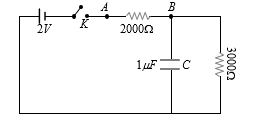CIrcuit questions
Question

# When the key K is pressed at time t=0 , which of the following statements about the current I in the resistor AB of the given circuit is trueEasy
Solution

## At time t = 0 i.e. when capacitor is charging, current  $\mathrm{i}=\frac{2}{2000}=1\mathrm{mA}$When capacitor is full charged, no current will pass through it, hence current through the circuit  $\mathrm{i}=\frac{2}{5000}=0.4\mathrm{mA}$

Get Instant Solutions